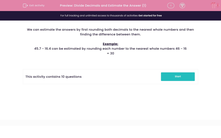# Divide Decimals and Estimate the Answer (1)

In this worksheet, students round two decimals to the nearest whole numbers before subtracting them.Key stage:  KS 2

Curriculum topic:   Maths and Numerical Reasoning

Curriculum subtopic:   Decimals

Difficulty level:#### Worksheet Overview

We can estimate the answers by first rounding both decimals to the nearest whole numbers and then finding the difference between them.

Example:

45.7 - 16.4 can be estimated by rounding each number to the nearest whole numbers 46 - 16

= 30

### What is EdPlace?

We're your National Curriculum aligned online education content provider helping each child succeed in English, maths and science from year 1 to GCSE. With an EdPlace account you’ll be able to track and measure progress, helping each child achieve their best. We build confidence and attainment by personalising each child’s learning at a level that suits them.

Get started••••